# Toroidal Network Topology

This sample was created in ConceptDraw DIAGRAM diagramming and vector drawing software using the Computer and Networks solution from Computer and Networks area of ConceptDraw Solution Park.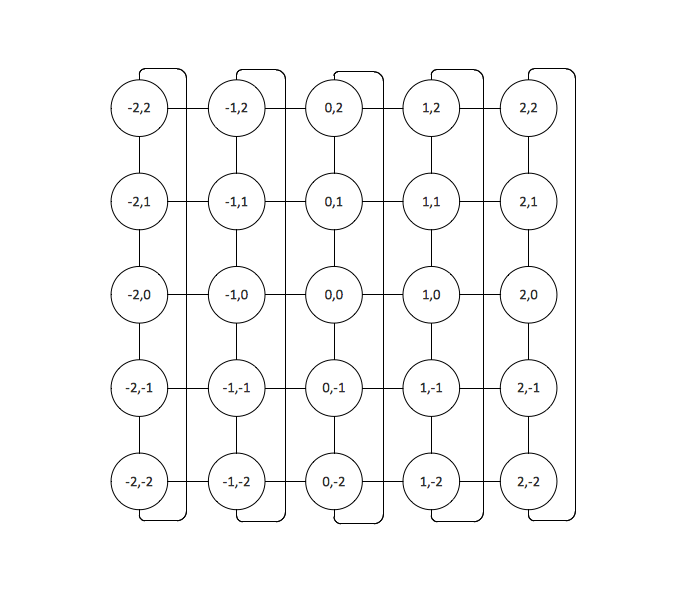Example 1. Toroidal Network Topology

This is example of the Toroidal network topology.

Network topology is the topological structure of the computer network.

Toroidal network topology is a kind of the grid network topology. The grid network topology is a topology in which the each node of the network is connected with two neighboring nodes along one or more dimensions. The Torus is a topology with n-dimensional grid network with circularly connection of the nodes. The network with Torus topology is named the Toroidal network.

Using the predesigned objects, templates and samples of the Computer and Networks Solution for ConceptDraw DIAGRAM you can create your own professional Computer Network Diagrams quick and easy.

The Computer Network Diagrams produced with ConceptDraw DIAGRAM are vector graphic documents and are available for reviewing, modifying, and converting to a variety of formats (image, HTML, PDF file, MS PowerPoint Presentation, Adobe Flash or MS Visio).

## See also Samples:

Related Solution:

### Hypercube Network Topology

This sample was created in ConceptDraw DIAGRAM v12 diagramming and vector drawing software using the Computer and Networks solution from Computer and Networks area of ConceptDraw Solution Park. This sample shows the Hypercube network topology. Network topology is the topological structure of the computer network. Hypercube is a type of the toroidal network. The Torus is a topology with n-dimensional grid network with circularly connection of the nodes. If the number of nodes along each dimension of the toroidal network is 2, it is a Hypercube network topology.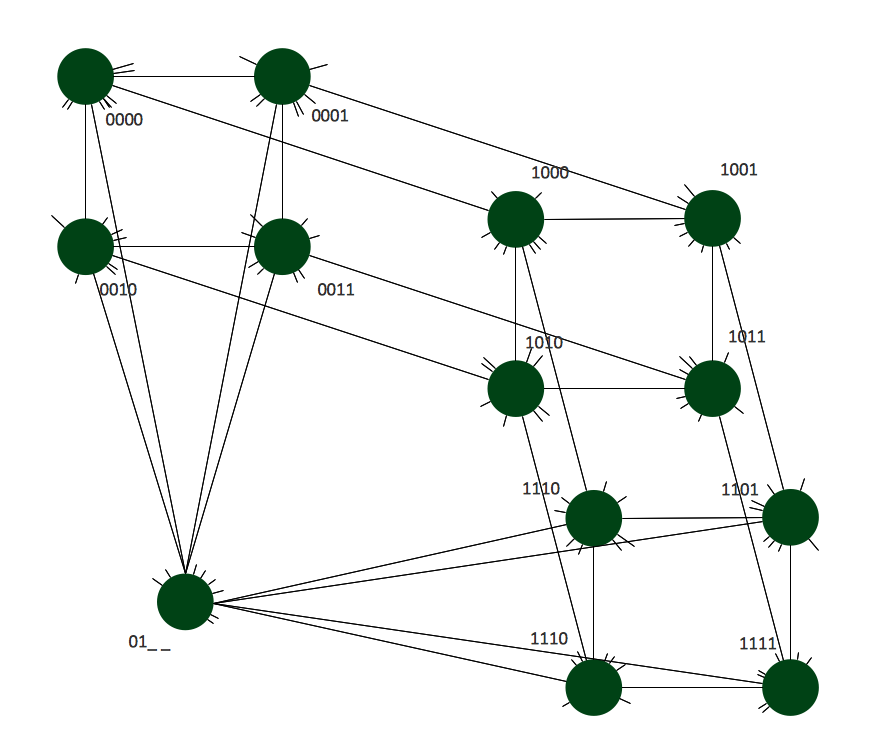Picture: Hypercube Network Topology
Related Solution:

### Point to Point Network Topology

This sample was created in ConceptDraw DIAGRAM v12 diagramming and vector drawing software using the Computer and Networks solution from Computer and Networks area of ConceptDraw Solution Park. This sample shows the Point-to-point network topology. Network topology is the topological structure of the computer network. Point-to-point network topology is a simple topology that displays the network of exactly two hosts (computers, servers, switches or routers) connected with a cable. Point-to-point topology is widely used in the computer networking and computer architecture.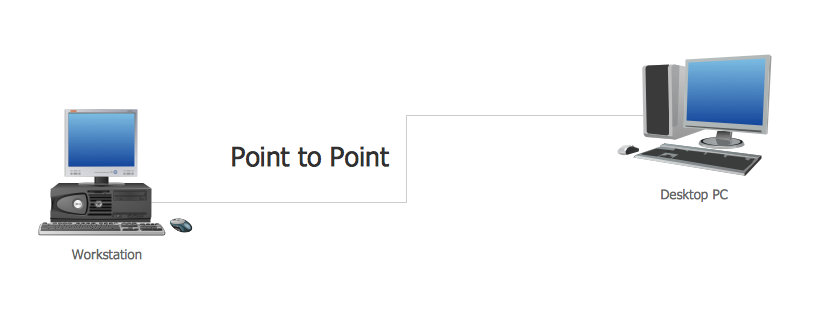Picture: Point to Point Network Topology
Related Solution:

### Hotel Network Topology Diagram

A network diagram represents the set of computers and network devices and the connections among them. This scheme can be developed for any institution or establishment. To illustrate this concept letâ€™s take for example, a hotel network topology diagram or a school network diagram. These diagrams depict access points, servers, workstations, firewalls and another equipment needed to provide a network. On this masterpiece drawing one will see a simple scheme a of connecting computers together. Such form of connecting can be applied for a hotel, guest house, bungalow, hut or something else. This diagram shows the images of the real LAN components. So, it represents a physical category of a network construction. It looks similar to a star - so this network configuration is named a star topology. The typical feature of this construction is a center point - usually it is hub, or router. The rays of this star means network connections. Computers, peripherals and other network details are placed on the ends of the star rays.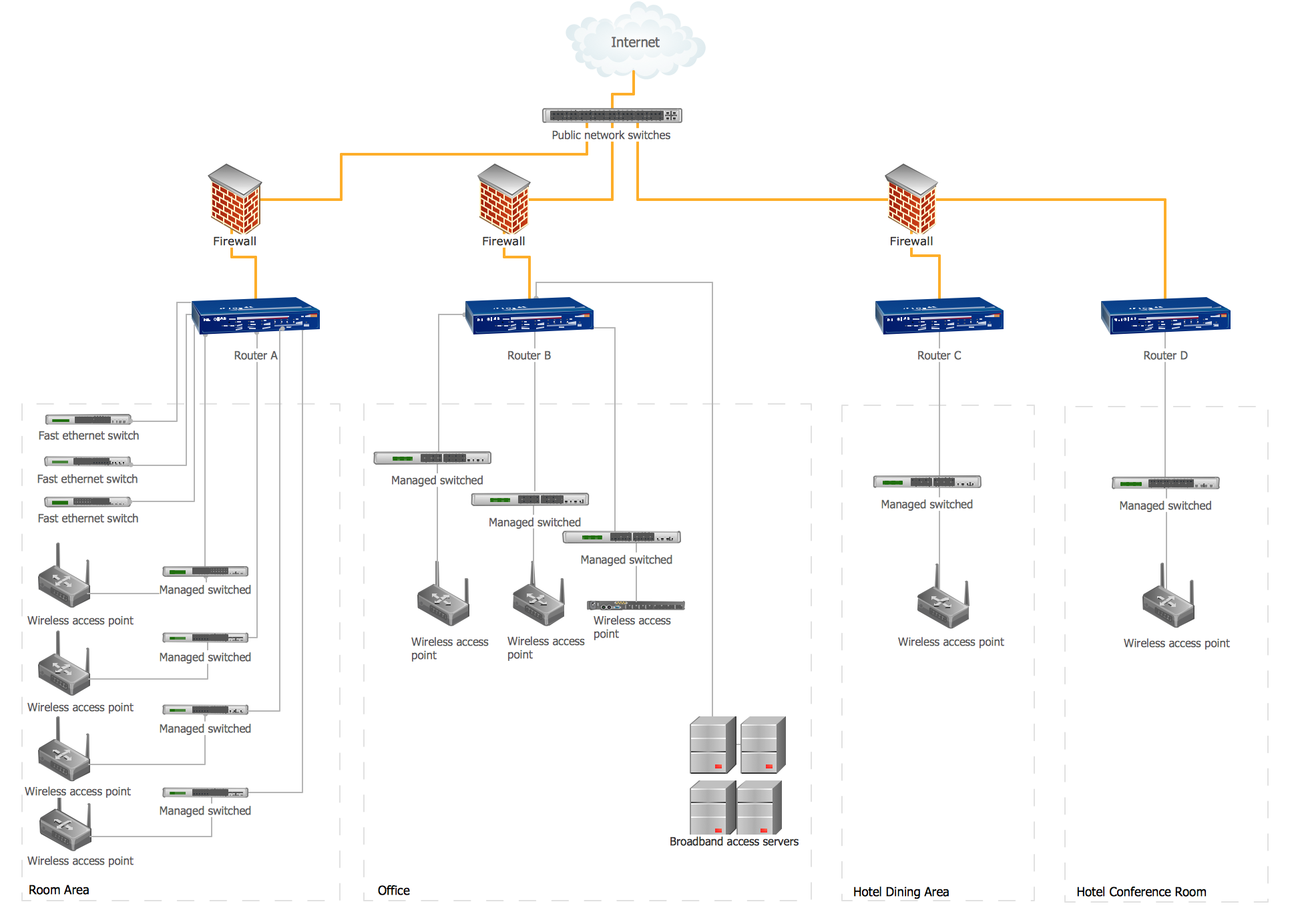Picture: Hotel Network Topology Diagram
Related Solution:

### How to Draw a Computer Network Diagrams

Planning a computer network can be a challenge for a junior specialist. However, knowing how to draw a computer network diagrams isnâ€™t a rocket science anymore. There are a lot of special software for creating such diagrams with predesigned templates and examples. The core for Network Fault Tolerance System presented here, is the equipment of Cisco. You can see here the certified Cisco equipment icons. Generally, ConceptDraw DIAGRAM v12 libraries contain more than half of a thousand objects representing the standardized images of Cisco equipment. ConceptDraw solution for network diagramming is a great network diagramming tool for any level skills - from students to network guru.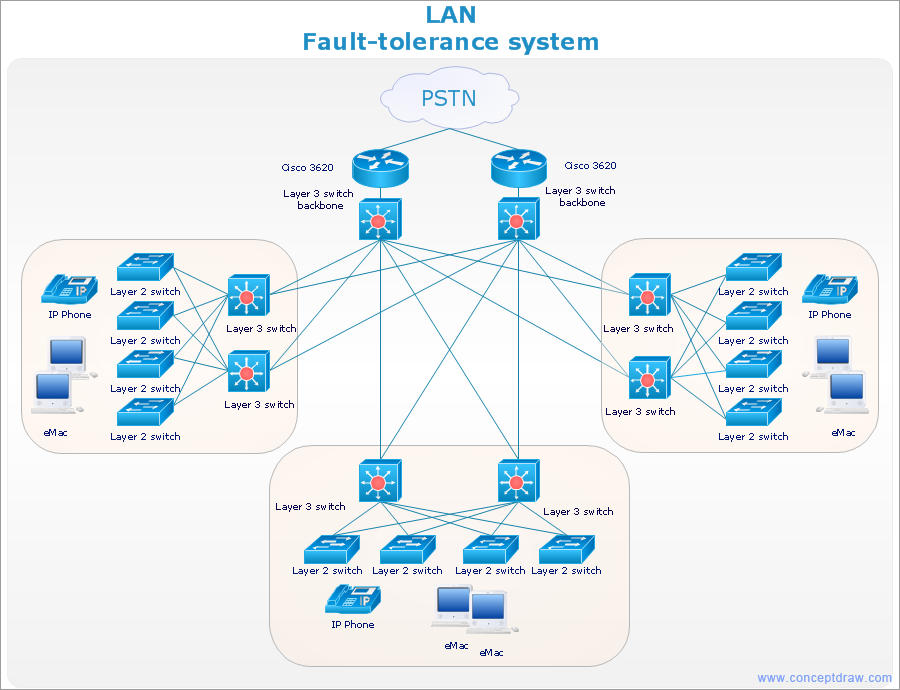Picture: How to Draw a Computer Network Diagrams
Related Solution:

### Star Network Topology

Nodes of any computer network are somehow organized in a hierarchy or a layout. Some of the common layouts like star network topology are more reliable and some like ring topology withstand high loads better. It is also important to distinguish logical topologies from physical. This diagram represents a typical view of the star network topology. The star network topology is one of the most frequently used network topologies in the majority of office and home networks. It is very popular because of its low cost and the easy maintenance. The plus of the star network topology is that if one computer on the local network is downed, this means that only the failed computer can not send or receive data. The other part of the network works normally. The minus of using star network topology is that all computers are connected to a single point-switch, or hub. Thus, if this equipment goes down, the whole local network comes down.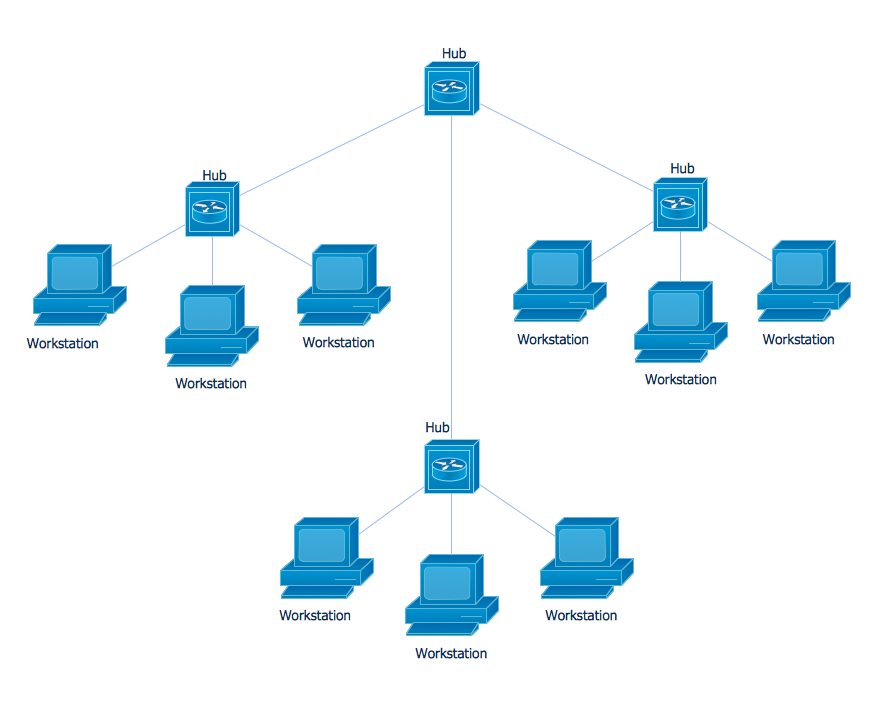Picture: Star Network Topology
Related Solution:

### Point-to-multipoint (P2MP) Network. Computer and Network Examples

A Point-to-multipoint (P2MP) communication is a communication that is accomplished through the one-to-many connection. This example was created in ConceptDraw DIAGRAM v12 using the Computer and Networks Area of ConceptDraw Solution Park and shows the Point-to-multipoint (P2MP) network.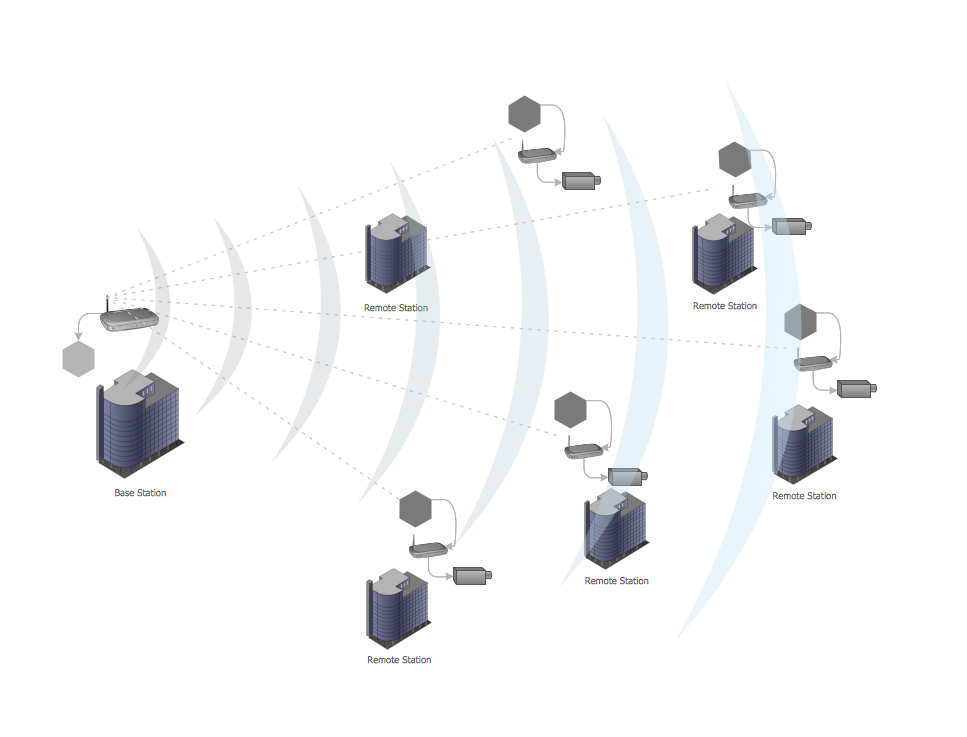Picture: Point-to-multipoint (P2MP) Network. Computer and Network Examples
Related Solution:

### Virtual networks. Computer and Network Examples

A Virtual network is a computer network that consists of virtual network links, i.e. between the computing devices there isn't a physical connection. Well known forms of network virtualization are virtual networks based on the virtual devices (for example the network based on the virtual devices inside a hypervisor), protocol-based virtual networks (VLAN, VPN, VPLS, Virtual Wireless network, etc.) and their combinations. This example was created in ConceptDraw DIAGRAM v12 using the Computer and Networks Area of ConceptDraw Solution Park and shows the Virtual network.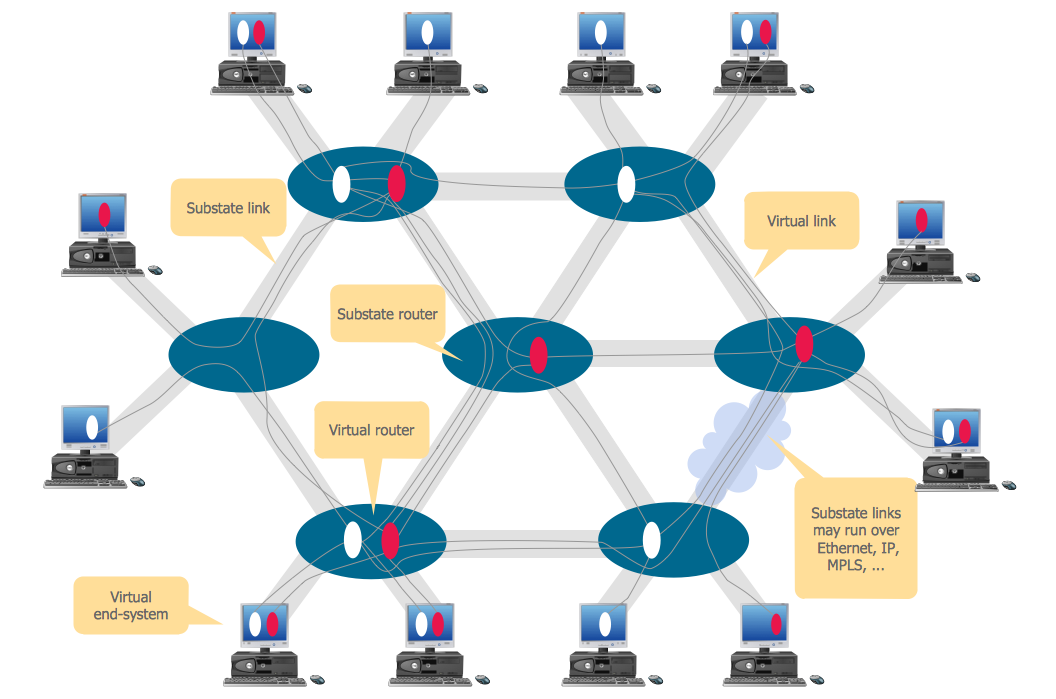Picture: Virtual networks. Computer and Network Examples
Related Solution:

### Complete Network Topology

This sample was created in ConceptDraw DIAGRAM v12 diagramming and vector drawing software using the Computer and Networks solution from Computer and Networks area of ConceptDraw Solution Park. A complete (fully connected) topology is a network topology in which there is a direct link between all pairs of nodes. In a fully connected network with n nodes, there are n(n-1)/2 direct links. Networks designed with this topology are usually very expensive to set up, but provide a high degree of reliability due to the multiple paths for data that are provided by the large number of redundant links between nodes.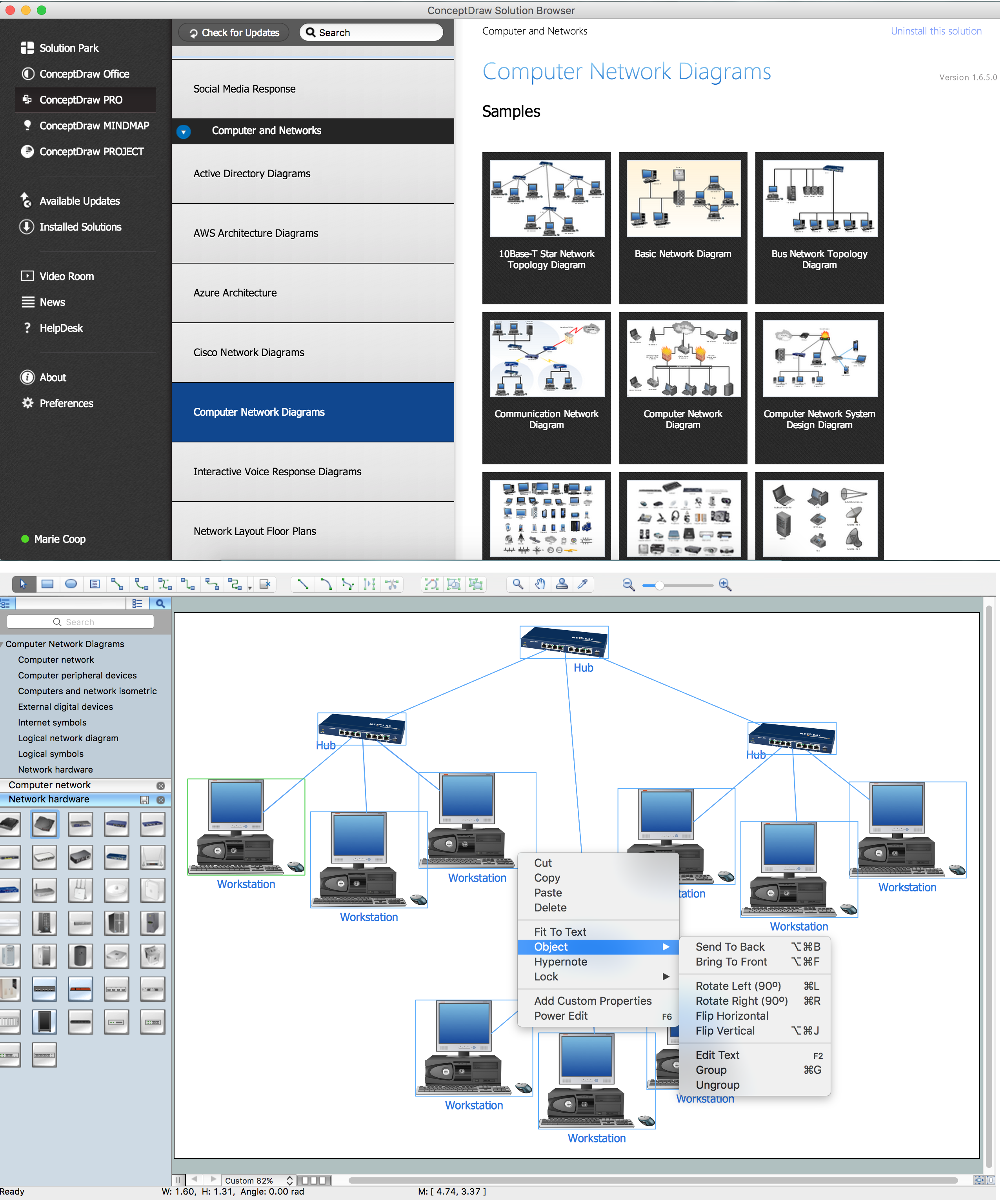Picture: Complete Network Topology
Related Solution: